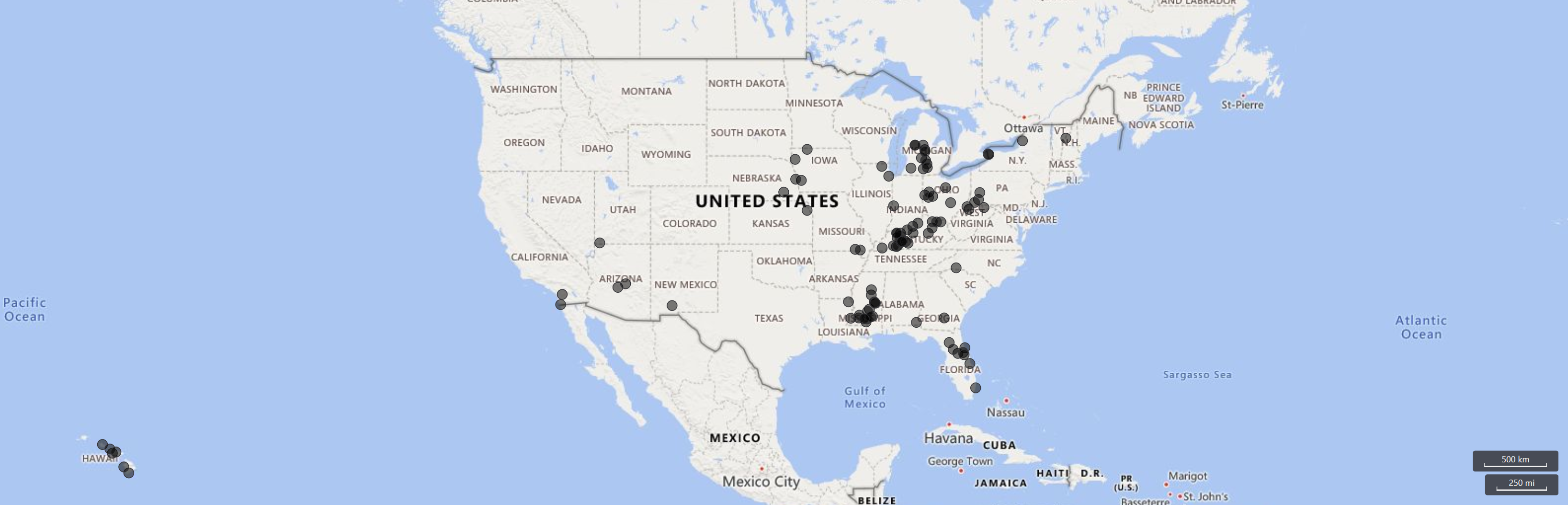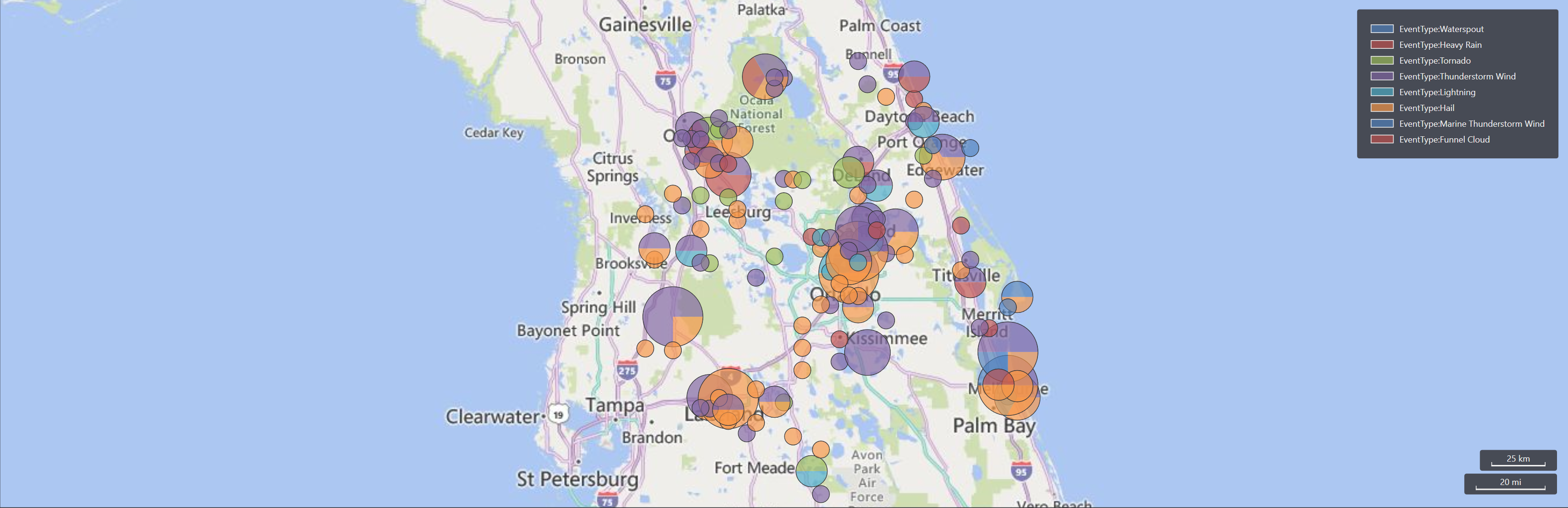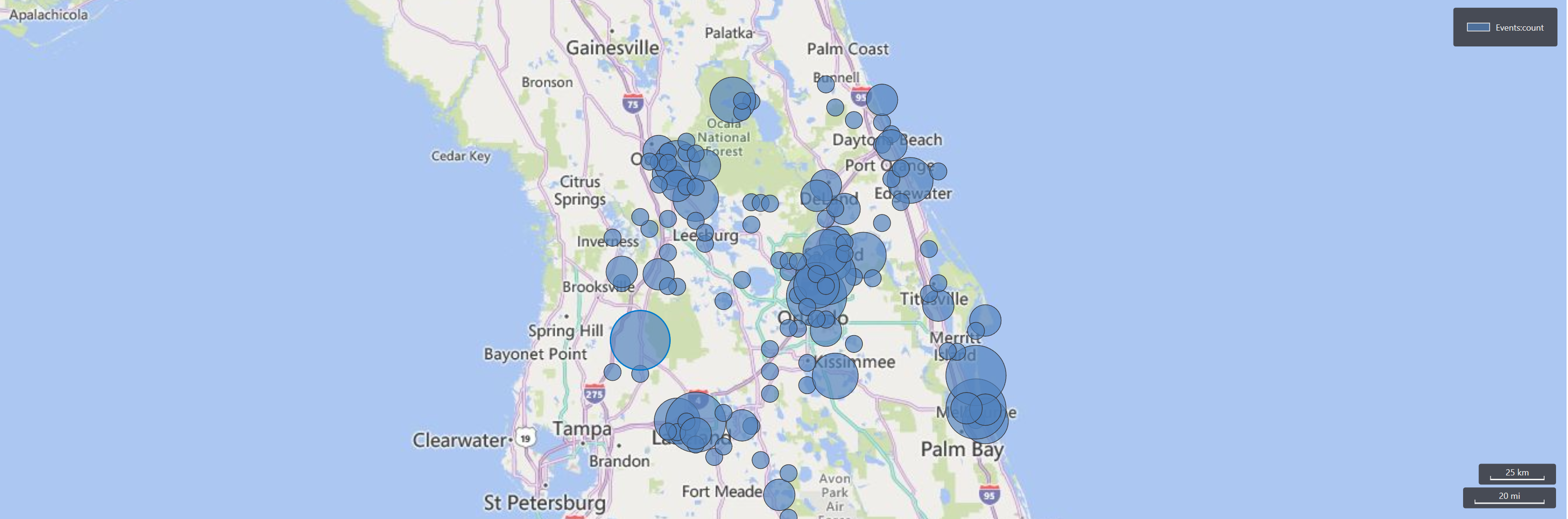# Geospatial visualizations

Geospatial data can be visualized using the render operator in Kusto Desktop Explorer or the Azure Data Explorer web UI. To download Kusto Desktop Explorer, see Kusto.Explorer installation and user interface.

## Visualize points on a map

It's possible to visualize points either using [Longitude, Latitude] columns, or GeoJSON column. The use of a series column is optional. Each point is defined by [Longitude, Latitude] pair, in that order.

### Example: Visualize points on a map

``````StormEvents
| take 100
| project BeginLon, BeginLat
| render scatterchart with (kind = map)
``````### Example: Visualize multiple series of points on a map

To visualize multiple series of points, each point is defined by a [Longitude, Latitude] pair, and the series is defined by a third column. In this example, the series is `EventType`.

``````StormEvents
| take 100
| project BeginLon, BeginLat, EventType
| render scatterchart with (kind = map)
``````### Example: Visualize series of points on data with multiple columns

If you have multiple columns in the result, you must specify the columns to be used for xcolumn (Longitude), ycolumn (Latitude), and series.

``````StormEvents
| take 100
| render scatterchart with (kind = map, xcolumn = BeginLon, ycolumns = BeginLat, series = EventType)
``````### Example: Visualize points on a map defined by GeoJSON dynamic values

In this example, the points are defined by GeoJSON dynamic values.

``````StormEvents
| project BeginLon, BeginLat
| summarize by hash=geo_point_to_s2cell(BeginLon, BeginLat, 5)
| project geo_s2cell_to_central_point(hash)
| render scatterchart with (kind = map)
``````## Visualization of pies or bubbles on a map

You can visualize pies or bubbles either using [Longitude, Latitude] columns or GeoJSON column. These visualizations can be created with color or numeric axes.

### Example: Visualize pie charts by location

The following example shows storm events aggregated by s2 cell. The chart aggregates events in pie charts by location.

``````StormEvents
| project BeginLon, BeginLat, EventType
| where geo_point_in_circle(BeginLon, BeginLat, real(-81.3891), 28.5346, 1000 * 100)
| summarize count() by EventType, hash = geo_point_to_s2cell(BeginLon, BeginLat)
| project geo_s2cell_to_central_point(hash), EventType, count_
| render piechart with (kind = map) // pie map rendering available only in Kusto Explorer desktop
``````### Example: Visualize bubbles using a color axis

The following example shows storm events aggregated by s2 cell. The chart aggregates events in bubble by location. The color-axis ("count") is the same for all events, and this is why bubbles are generated by the `render` operator.

``````StormEvents
| project BeginLon, BeginLat, EventType
| where geo_point_in_circle(BeginLon, BeginLat, real(-81.3891), 28.5346, 1000 * 100)
| summarize count() by EventType, hash = geo_point_to_s2cell(BeginLon, BeginLat)
| project geo_s2cell_to_central_point(hash), count_
| extend Events = "count"
| render piechart with (kind = map)
``````